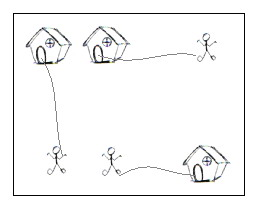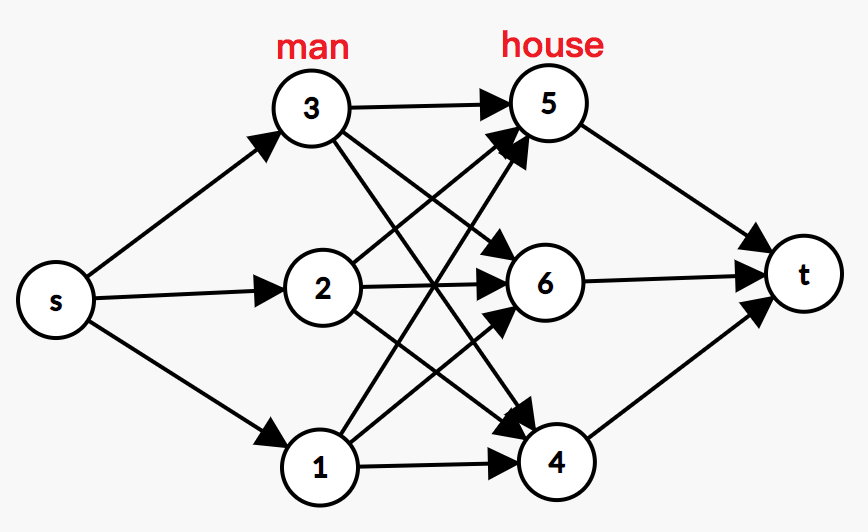# POJ2195 Going Home（最小费用最大流）

## Going Home

• Time Limit: 1000MS
• Memory Limit: 65536K
• Total Submissions: 24963
• Accepted: 12509

### Description

On a grid map there are n little men and n houses. In each unit time, every little man can move one unit step, either horizontally, or vertically, to an adjacent point. For each little man, you need to pay a \$1 travel fee for every step he moves, until he enters a house. The task is complicated with the restriction that each house can accommodate only one little man.

Your task is to compute the minimum amount of money you need to pay in order to send these n little men into those n different houses. The input is a map of the scenario, a '.' means an empty space, an 'H' represents a house on that point, and am 'm' indicates there is a little man on that point.You can think of each point on the grid map as a quite large square, so it can hold n little men at the same time; also, it is okay if a little man steps on a grid with a house without entering that house.

### Input

There are one or more test cases in the input. Each case starts with a line giving two integers N and M, where N is the number of rows of the map, and M is the number of columns. The rest of the input will be N lines describing the map. You may assume both N and M are between 2 and 100, inclusive. There will be the same number of 'H's and 'm's on the map; and there will be at most 100 houses. Input will terminate with 0 0 for N and M.

### Output

For each test case, output one line with the single integer, which is the minimum amount, in dollars, you need to pay.

### Sample Input

2 2
.m
H.
5 5
HH..m
.....
.....
.....
mm..H
7 8
...H....
...H....
...H....
mmmHmmmm
...H....
...H....
...H....
0 0

### Sample Output

2
10
28

### Source

Pacific Northwest 2004

### 链接

http://poj.org/problem?id=2195

### 题意

n个小人回到n间房子，一对一，现要使移动的总距离最少。

### 题解### 代码

StatusAccepted
Time141ms
Memory800kB
Length3412
#include <iostream>
#include <cstdio>
#include <cstring>
#include <algorithm>
#include <queue>
using namespace std;
const int maxn = 10010; // 点数
const int maxm = 100010; // 边数
const int inf = 0x3f3f3f3f;

char map[maxn][maxn];
struct Node
{
int x, y;
}nodem[maxn], nodeh[maxn];

struct Edge
{
int to, next, cap, flow, cost;
}edge[maxm];

int n, m, tot;
bool vis[maxn];

void init()
{
tot = 0;
}

void addedge(int u, int v, int cap, int cost) // 链式前向星存图
{
edge[tot].to = v;
edge[tot].cap = cap;
edge[tot].cost = cost;
edge[tot].flow = 0;
}

bool spfa(int s, int t) // spfa寻找最小费用增广路
{
queue <int> Q;
for (int i = 0; i <= n; ++i)
{
dis[i] = inf;
vis[i] = false;
pre[i] = -1;
}
dis[s] = 0;
vis[s] = true;
Q.push(s);
while (!Q.empty())
{
int u = Q.front();
Q.pop();
vis[u] = false;
for (int i = head[u]; i != -1; i = edge[i].next)
{
int v = edge[i].to;
if (edge[i].cap > edge[i].flow && dis[v] > dis[u] + edge[i].cost)
{
dis[v] = dis[u] + edge[i].cost;
pre[v] = i;
if (!vis[v])
{
vis[v] = true;
Q.push(v);
}
}
}
}
if (pre[t] == -1)
return false;
return true;
}

int minCostMaxflow(int s, int t, int &mincost) // 计算费用
{
int maxflow = 0;
mincost = 0;
while (spfa(s, t))
{
int Min = inf;
for (int i = pre[t]; i != -1; i = pre[edge[i^1].to]) // 每次增加的流量
Min = min(Min, edge[i].cap - edge[i].flow);
for (int i = pre[t]; i != -1; i = pre[edge[i^1].to])
{
edge[i].flow += Min;
edge[i^1].flow -= Min;
mincost += edge[i].cost * Min;
}
maxflow += Min;
}
return maxflow;
}

int main()
{
int r, c, cost, cap = 1, s, t;
while (scanf("%d%d", &r, &c) == 2 && r && c)
{
init();
int cntm = 0, cnth = 0;
for (int i = 1; i <= r; ++i)
{
scanf("%s", map[i] + 1);
for (int j = 1; j <= c; ++j)
{
if (map[i][j] == 'm') // 记录小人
nodem[++cntm].x = i, nodem[cntm].y = j;
else if (map[i][j] == 'H') // 记录房子
nodeh[++cnth].x = i, nodeh[cnth].y = j;
}
}
//        cout << cntm << " " << cnth << endl; // 这个一开始多了1
for (int i = 1; i <= cntm; ++i) // 小人与房子之间建立一条容量为1费用为最短移动距离的边
{
for (int j = 1; j <= cnth; ++j)
{
cost = abs(nodem[i].x - nodeh[j].x) + abs(nodem[i].y - nodeh[j].y);
addedge(i, cntm + j, cap, cost);
addedge(cntm + j, i, 0, -cost);
}
}
s = 0, n = cntm + cnth + 1, t = cnth + cntm + 1;
for (int i = 1; i <= cntm; ++i) // 源点与小人之间建边，容量为1，费用为0
{
}
for (int i = 1; i <= cnth; ++i) // 房子与汇点之间建边，容量为1，费用为0
{
addedge(cntm + i, t, cap, 0);
addedge(t, cntm + i, 0, 0);
}
int mincost = 0;
minCostMaxflow(s, t, mincost); // 求解最小费用最大流
cout << mincost << endl;
}
return 0;
}

The end.
2018-07-31 星期二